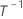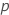# Pareto chart

The reference line for statistical significance on the Pareto chart is drawn at t, where t is the (1 - α / 2) quantile of a t-distribution with degrees of freedom equal to the degrees of freedom for the error term.

The calculation of the standardized effect depends on the degrees of freedom for the term. Continuous predictors and 2-level categorical predictors have 1 degree of freedom. For terms with 1 degree of freedom, the standardized effect is the absolute value of the t-statistic for the coefficient in the Coefficients table.

Categorical predictors with 3 or more levels and their interactions have more than 1 degree of freedom. For terms with more than 1 degree of freedom, the standardized effect has the following form:

## Notation

TermDescriptionthe inverse cumulative distribution function from a t-distribution with degrees of freedom equal to the degrees of freedom for errorthe p-value from the ANOVA table for the term
By using this site you agree to the use of cookies for analytics and personalized content.  Read our policy